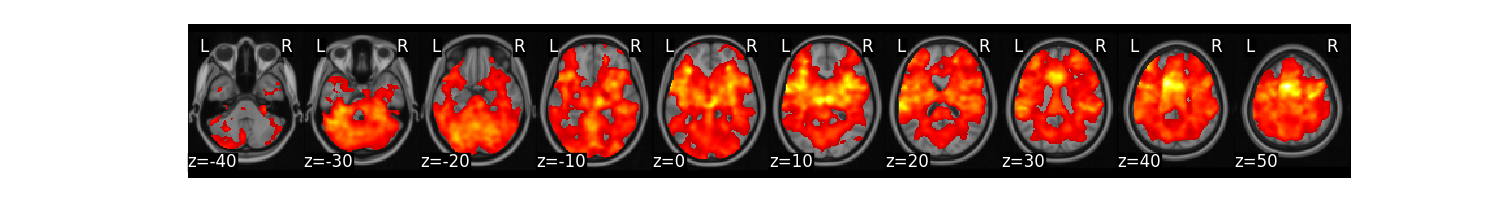# Univariate Regression¶

This example simulates data according to a very simple sketch of brain imaging data and applies a standard two-level univariate GLM to identify significant voxels.

Here we fetch the pain dataset used in Chang et al., 2015. In this dataset there are 28 subjects with 3 separate beta images reflecting varying intensities of thermal pain (i.e., high, medium, low). The data will be downloaded to ~/nilearn_data, and automatically loaded as a Brain_Data() instance. The metadata will be stored in data.X.

```from nltools.datasets import fetch_pain

data = fetch_pain()
```
```/usr/share/miniconda3/envs/test/lib/python3.8/site-packages/nilearn/maskers/nifti_masker.py:108: UserWarning: imgs are being resampled to the mask_img resolution. This process is memory intensive. You might want to provide a target_affine that is equal to the affine of the imgs or resample the mask beforehand to save memory and computation time.
warnings.warn(
```

## Run Univariate Regression¶

We can loop over subjects and predict the intensity of each voxel from a simple model of pain intensity and an intercept. This is just for illustration purposes as there are only 3 observations per subject. We initialize an empty Brain_Data() instance and loop over all subjects running a univariate regression separately for each participant. We aggregate the beta estimates for pain intensity across subjects.

```from nltools.data import Brain_Data
import numpy as np
import pandas as pd

all_sub = Brain_Data()
for s in subject_id.unique():
sdat.X = pd.DataFrame(data={'Intercept':np.ones(sdat.shape()),'Pain':sdat.X['PainLevel']})
stats = sdat.regress()
all_sub = all_sub.append(stats['beta'])
```

We can now run a one-sample t-test at every voxel to test whether it is significantly different from zero across participants. We will threshold the results using FDR correction, q < 0.001.

```t_stats = all_sub.ttest(threshold_dict={'fdr':.001})
t_stats['thr_t'].plot()
``````/usr/share/miniconda3/envs/test/lib/python3.8/site-packages/nilearn/masking.py:974: UserWarning: Data array used to create a new image contains 64-bit ints. This is likely due to creating the array with numpy and passing `int` as the `dtype`. Many tools such as FSL and SPM cannot deal with int64 in Nifti images, so for compatibility the data has been converted to int32.
```

## Run Linear Contrast¶

Obviously, the univariate regression isn’t a great idea when there are only three observations per subject. As we predict a monotonic increase in pain across pain intensities, we can also calculate a linear contrast c=(-1,0,1). This is simple using matrix multiplication on the centered pain intensity values.

```all_sub = []
for sub in subject_id.unique():
sdat.X = pd.DataFrame(data={'Pain':sdat.X['PainLevel']})
all_sub.append(sdat * np.array(sdat.X['Pain'] - 2))
all_sub = Brain_Data(all_sub)
```

We can again run a one-sample t-test at every voxel using an FDR threshold of q < 0.001.

```t_stats = all_sub.ttest(threshold_dict={'fdr':.001})
t_stats['thr_t'].plot()
``````/usr/share/miniconda3/envs/test/lib/python3.8/site-packages/nilearn/masking.py:974: UserWarning: Data array used to create a new image contains 64-bit ints. This is likely due to creating the array with numpy and passing `int` as the `dtype`. Many tools such as FSL and SPM cannot deal with int64 in Nifti images, so for compatibility the data has been converted to int32.